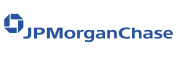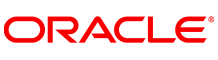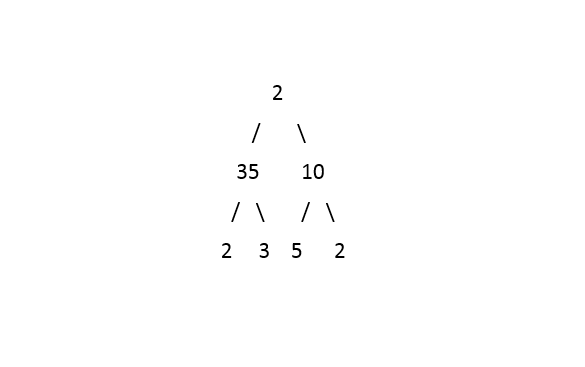New update is available. Click here to update.
Topics

# Left View Of Binary Tree

Moderate0/80
Average time to solve is 30m+56 more companies

## Problem statement

You have been given a Binary Tree of 'n' nodes, where the nodes have integer values

Example :
``````If the input tree is as depicted in the picture:
````````````The Left View of the tree will be:  2 35 2
``````
Detailed explanation ( Input/output format, Notes, Images )
Sample Input 1 :
``````2 35 10 2 3 5 2 -1 -1 -1 -1 -1 -1 -1 -1 -1 -1
``````
Sample Output 1 :
``````2 35 2
``````
Explanation of Sample Input 1 :
``````The test case is explained in the problem statement.
``````
Sample Input 2 :
``````1 2 3 4 5 -1 7 -1 -1 -1 -1 -1 -1
``````
Sample Output 2 :
``````1 2 4
``````
Explanation of Sample Input 2 :
``````The Tree looks as follows:
1
/   \
2     3
/   \     \
4     5     7
``````
Expected time complexity:
``````The expected time complexity is O(n).
``````
Constraints :
``````0 <= 'n' <= 10^5
1 <= 'data' <= 10^5

Where ‘n’ is the total number of nodes in the binary tree, and 'data' is the value of the binary tree node.

Time limit: 1sec
``````Console